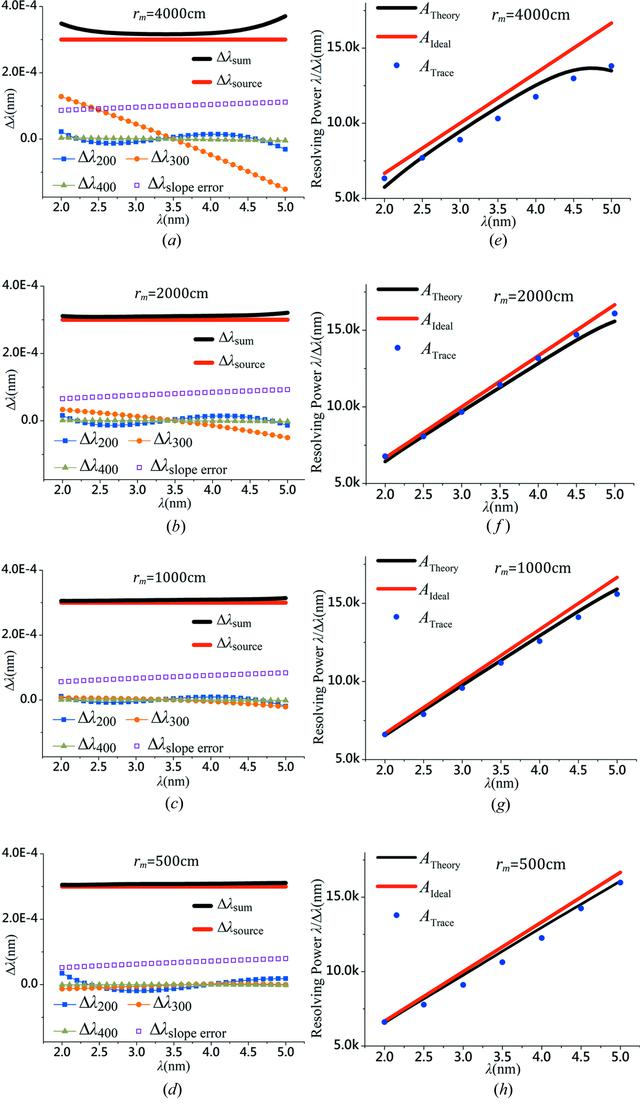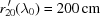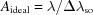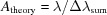disable zoom     view article Figure 6 The simulated results of the major factors which influence the resolving power of the spectrometer, including the source size (thick red line), the optical fabrication error (empty squares), the optical aberrations, i.e. defocus (filled squares), coma (filled circles), spherical aberration (gray triangles) and overall (thick black lines). The results for various object distances are presented, (a) rm = 4000 cm, (b) rm = 2000  cm, (c) rm = 1000 cm and (d)  rm = 500 cm; and the image distances for all four cases are identical:. The corresponding resolving powers of (a)–(d) are calculated and presented in (e)–(h), respectively, where for each case three types of the spectral resolutions are shown:,and Atrace (obtained from the ray-tracing program, see Fig. 7), for (e) rm = 4000 cm, (f) rm = 2000 cm, (g) rm = 1000 cm and (h) rm = 500 cm.JOURNAL OFSYNCHROTRONRADIATION
ISSN: 1600-5775
Volume 25| Part 3| May 2018| Pages 738-747